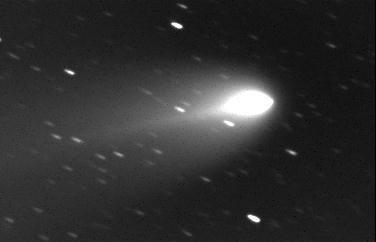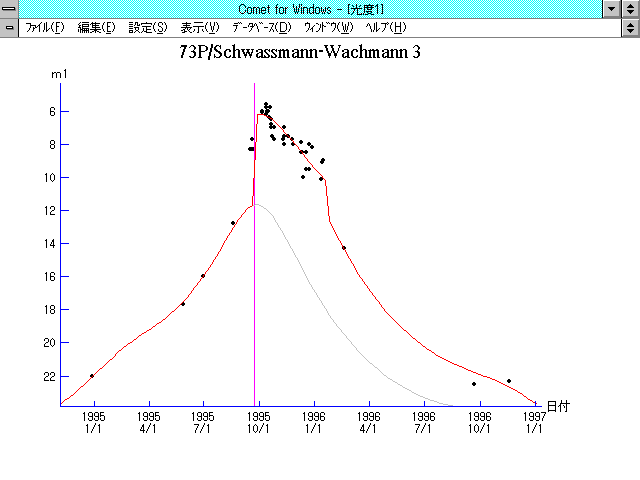# 73P/Schwassmann-Wachmann 3 (1995)###Pictures###Orbital Elements

```   The following improved orbital elements by Kenji Muraoka, are
from 133 observations 1995 Oct. 7 to 1996 Nov. 19, perturbations
by 9 Planets and 5 minor planets were taken into account.
The mean residual is +/- 2.08 arc seconds.

Epoch  =  1995 Oct.  10.0  TT      JDT = 2450000.5
T  =  1995 Sept. 22.87476      +/- 0.00064 (m.e.) TT
Peri. =  198.74610                +/- 0.00157
Node  =   69.94844                +/- 0.00182   (2000.0)
Incl. =   11.42388                +/- 0.00050
q  =    0.9326558              +/- 0.0000279 AU
e  =    0.6947507              +/- 0.0000052
a  =    3.0553907              +/- 0.0000753 AU
n  =    0.18454571             +/- 0.00000683
P  =    5.341                  +/- 0.0001976  years
(+/- 0.07 day)

Nuclear C

The following improved orbital elements, by Kenji Muraoka, are from
226 observations 1989 to 1996, including 9 Planets, Moon and 5 minor
planets perturbations and non-gravitational effect of style II.
The mean residual is  +/- 0.89 arc seconds.

Epoch  =  1995 Oct.  10.0  TT      JDT = 2450000.5
T  =  1995 Sept. 22.88978      +/- 0.00006 (m.e.) TT
Peri. =  198.76927                +/- 0.00017
Node  =   69.94661                +/- 0.00015   (2000.0)
Incl. =   11.42389                +/- 0.00003
q  =    0.9327815              +/- 0.0000005 AU
e  =    0.6948482              +/- 0.0000008
a  =    3.0567786              +/- 0.0000027 AU
n  =    0.18442004             +/- 0.00000025
P  =    5.344                  +/- 0.0000071  years
A1  =   +0.923                  +/- 0.016
A2  =   +0.21829                +/- 0.00439
A3  =   -0.204                  +/- 0.009

Nuclear C

The following improved orbital elements, by Kenji Muraoka, are
from 191 observations 1994 Dec. 28 to 1996 Nov. 19, perturbations
by 9 Planets, Moon and 5 minor planets were taken into account.
The mean residual is +/- 0.79 arc seconds.

Epoch  =  1995 Oct.  10.0  TT      JDT = 2450000.5
T  =  1995 Sept. 22.89089      +/- 0.00004 (m.e.) TT
Peri. =  198.76836                +/- 0.00019
Node  =   69.94839                +/- 0.00019   (2000.0)
Incl. =   11.42387                +/- 0.00003
q  =    0.9327852              +/- 0.0000026 AU
e  =    0.6948979              +/- 0.0000007
a  =    3.0572892              +/- 0.0000055 AU
n  =    0.18437384             +/- 0.00000049
P  =    5.346                  +/- 0.0000143  years
(+/- 0.01 day)
```

###Finding Charts1995 Jan. 3 - 1995 Nov. 291995 Oct. 10 - 1996 Dec. 3

###Magnitudes Graph

```        m1 = 11.5 + 5 log d + 18.0 log r  [   ,  0]  (             - 1995 Sept.22)
m1 =  5.8 + 5 log d + 10.0 log r  [  0,120]  (1995 Sept.22 - 1996 Jan. 20)
m1 =  2.5 + 5 log d + 30.0 log r  [120,   ]  (1996 Jan. 20 -             )

* Gray curve is:  m1 = 11.5 + 5 log d + 18.0 log r
```##### The orbital elements are calculated by Kenji Muraoka. The charts are made with StellaNavigator Ver.2.0 for Windows (AstroArts / ASCII). The magnitudes graphs are made with Comet for Windows.